Скачать презентацию CH 3 Deadlock When 2 or more processes

7ecf7c588ee9ab522ab2af7cab2088e8.ppt

• Количество слайдов: 44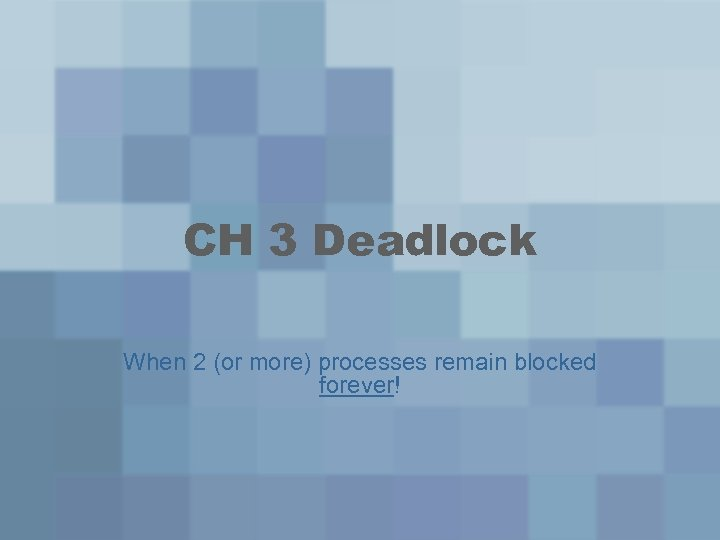CH 3 Deadlock When 2 (or more) processes remain blocked forever!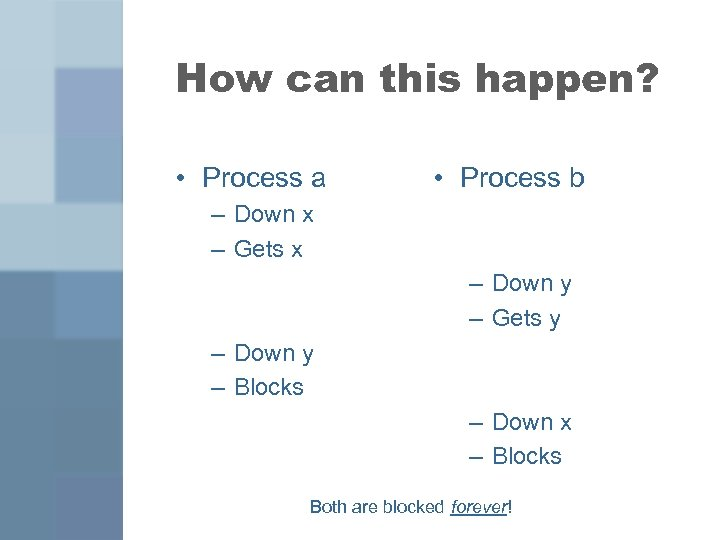How can this happen? • Process a • Process b – Down x – Gets x – Down y – Gets y – Down y – Blocks – Down x – Blocks Both are blocked forever!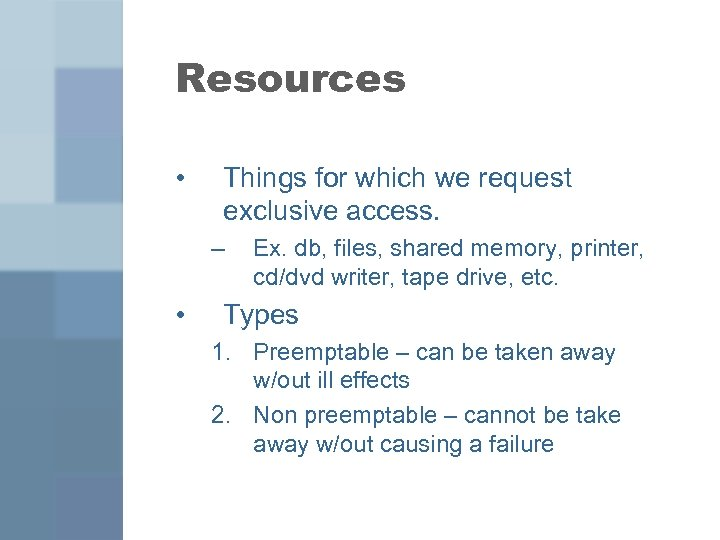Resources • Things for which we request exclusive access. – • Ex. db, files, shared memory, printer, cd/dvd writer, tape drive, etc. Types 1. Preemptable – can be taken away w/out ill effects 2. Non preemptable – cannot be take away w/out causing a failure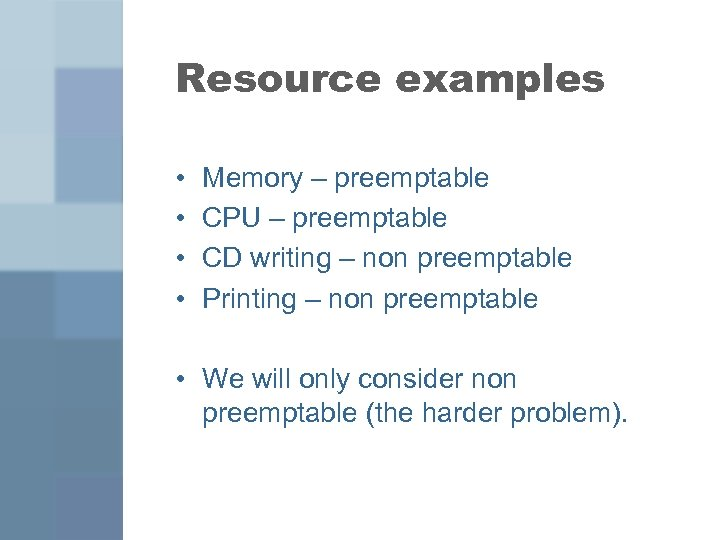Resource examples • • Memory – preemptable CPU – preemptable CD writing – non preemptable Printing – non preemptable • We will only consider non preemptable (the harder problem).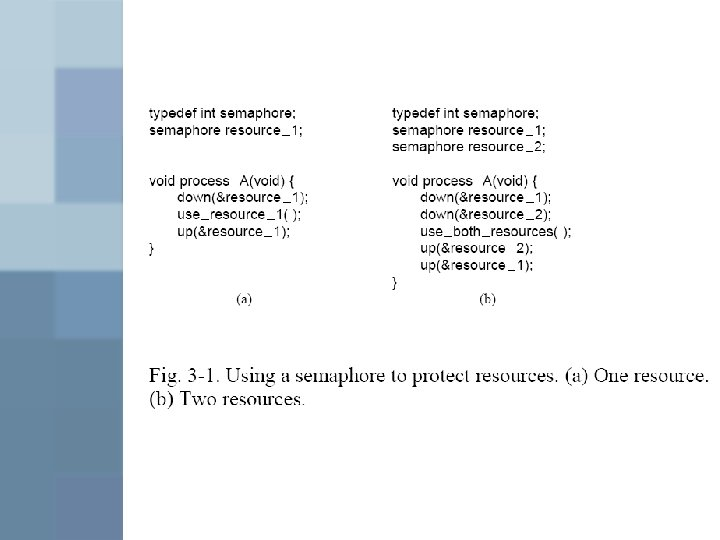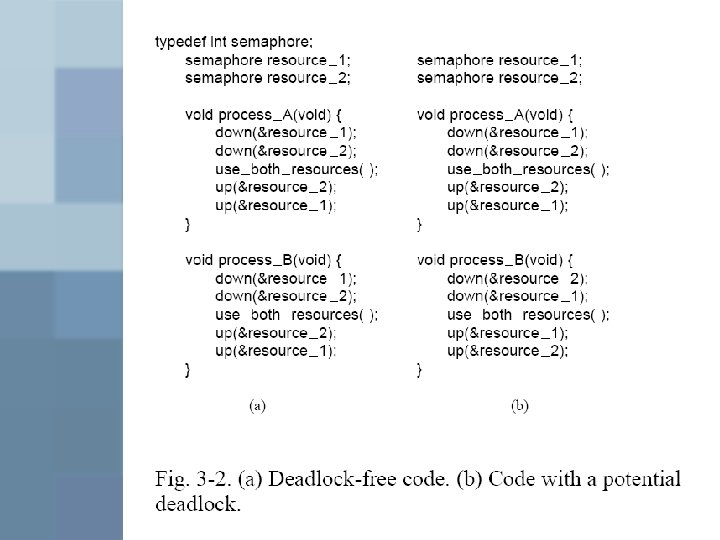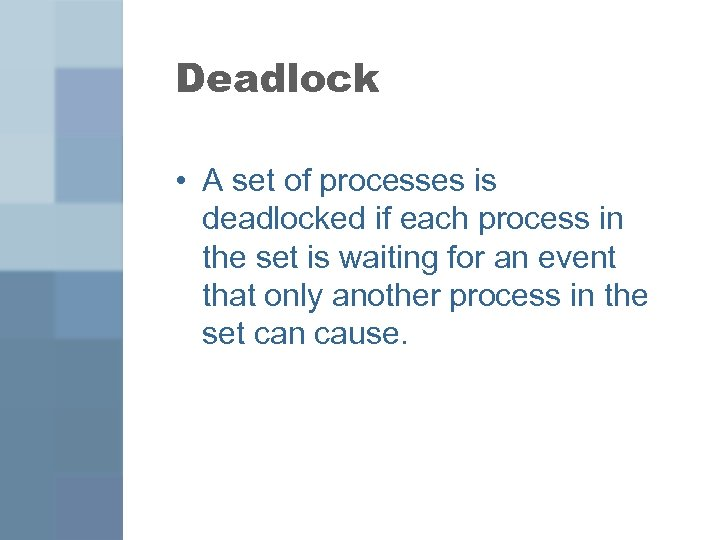Deadlock • A set of processes is deadlocked if each process in the set is waiting for an event that only another process in the set can cause.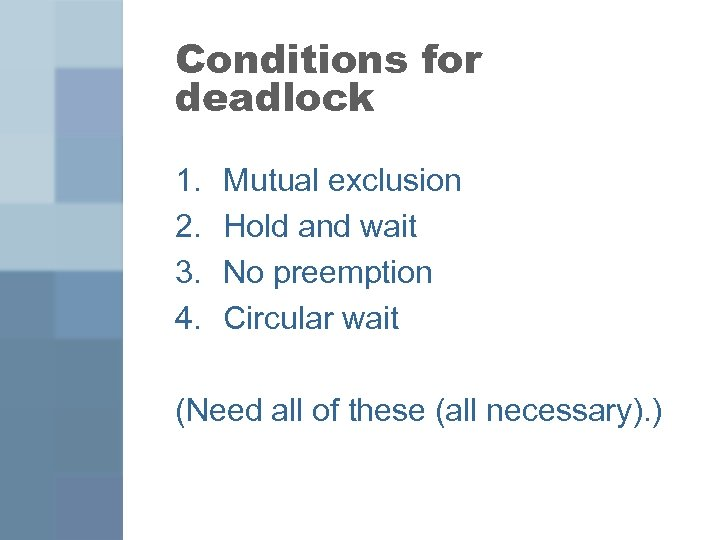Conditions for deadlock 1. 2. 3. 4. Mutual exclusion Hold and wait No preemption Circular wait (Need all of these (all necessary). )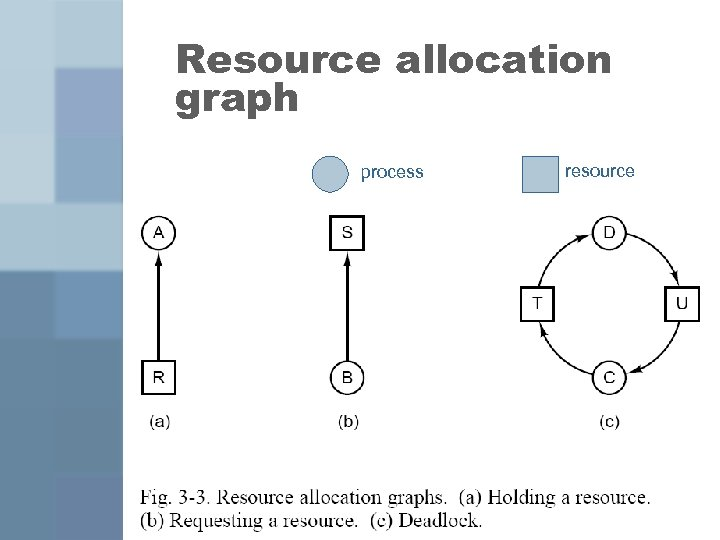Resource allocation graph process resource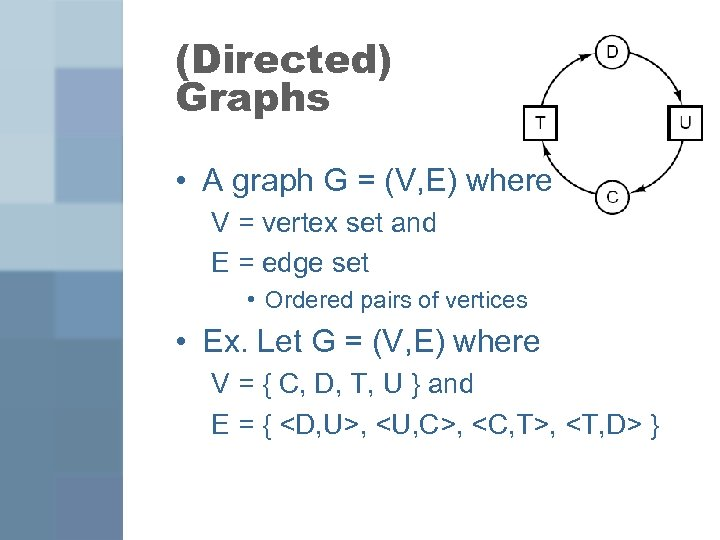(Directed) Graphs • A graph G = (V, E) where V = vertex set and E = edge set • Ordered pairs of vertices • Ex. Let G = (V, E) where V = { C, D, T, U } and E = { , , , }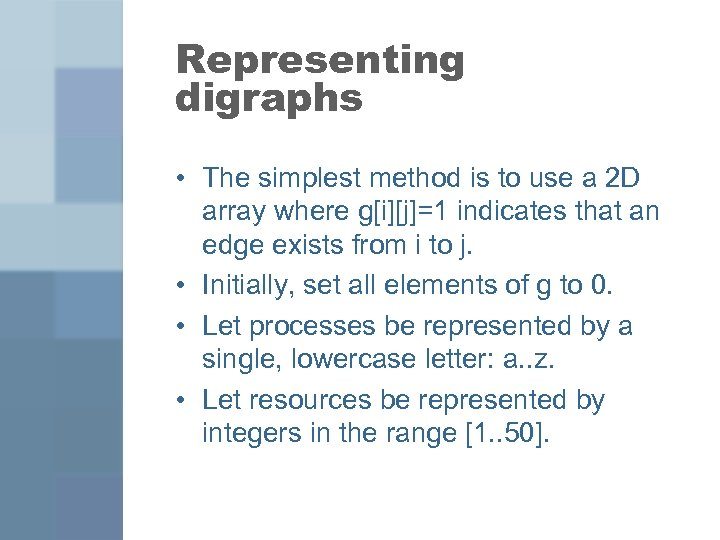Representing digraphs • The simplest method is to use a 2 D array where g[i][j]=1 indicates that an edge exists from i to j. • Initially, set all elements of g to 0. • Let processes be represented by a single, lowercase letter: a. . z. • Let resources be represented by integers in the range [1. . 50].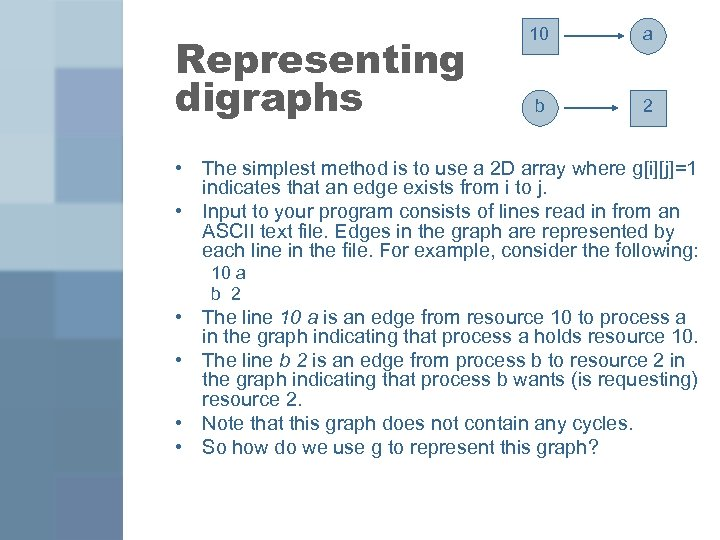Representing digraphs 10 a b 2 • The simplest method is to use a 2 D array where g[i][j]=1 indicates that an edge exists from i to j. • Input to your program consists of lines read in from an ASCII text file. Edges in the graph are represented by each line in the file. For example, consider the following: 10 a b 2 • The line 10 a is an edge from resource 10 to process a in the graph indicating that process a holds resource 10. • The line b 2 is an edge from process b to resource 2 in the graph indicating that process b wants (is requesting) resource 2. • Note that this graph does not contain any cycles. • So how do we use g to represent this graph?Representing digraphs 10 a b 2 • The simplest method is to use a 2 D array where g[i][j]=1 indicates that an edge exists from i to j. • Input to your program consists of lines read in from an ASCII text file. Edges in the graph are represented by each line in the file. For example, consider the following: 10 a b 2 • The line 10 a is an edge from resource 10 to process a in the graph indicating that process a holds resource 10. • The line b 2 is an edge from process b to resource 2 in the graph indicating that process b wants (is requesting) resource 2. • Note that this graph does not contain any cycles. • So how do we use g to represent this graph? g[‘a’] = 1; g[‘b’] = 1;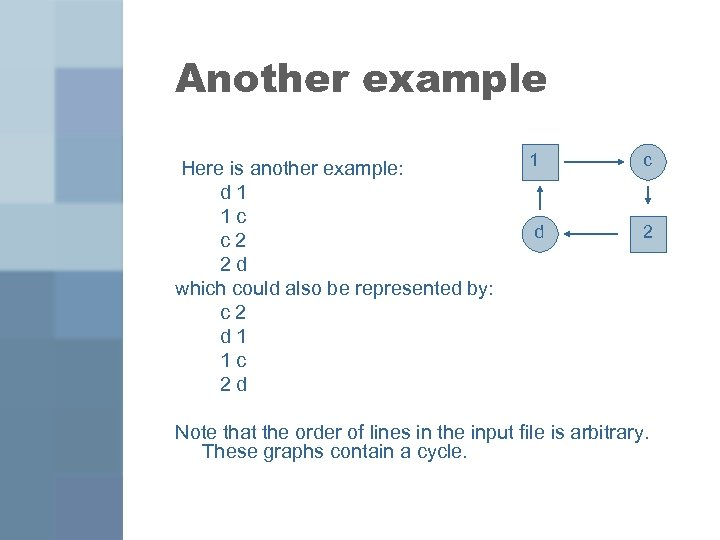Another example Here is another example: d 1 1 c c 2 2 d which could also be represented by: c 2 d 1 1 c 2 d 1 d c 2 Note that the order of lines in the input file is arbitrary. These graphs contain a cycle.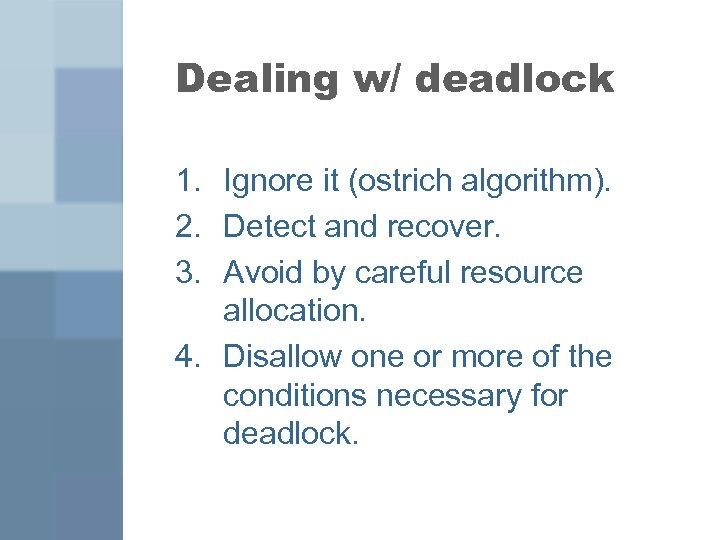Dealing w/ deadlock 1. Ignore it (ostrich algorithm). 2. Detect and recover. 3. Avoid by careful resource allocation. 4. Disallow one or more of the conditions necessary for deadlock.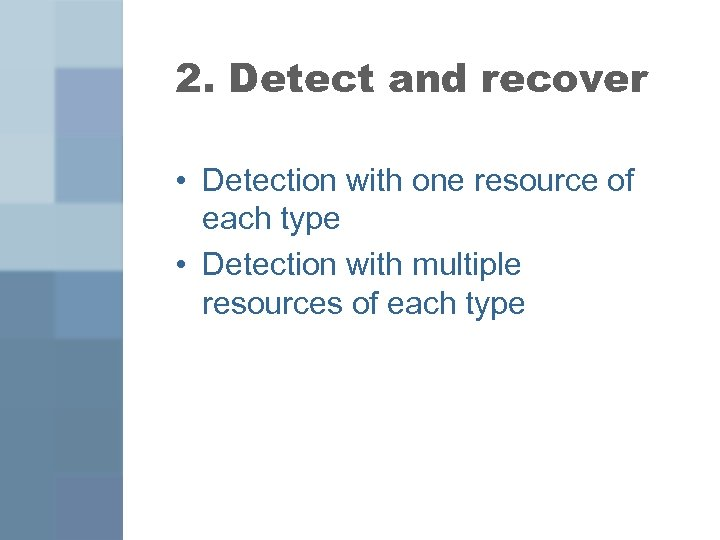2. Detect and recover • Detection with one resource of each type • Detection with multiple resources of each type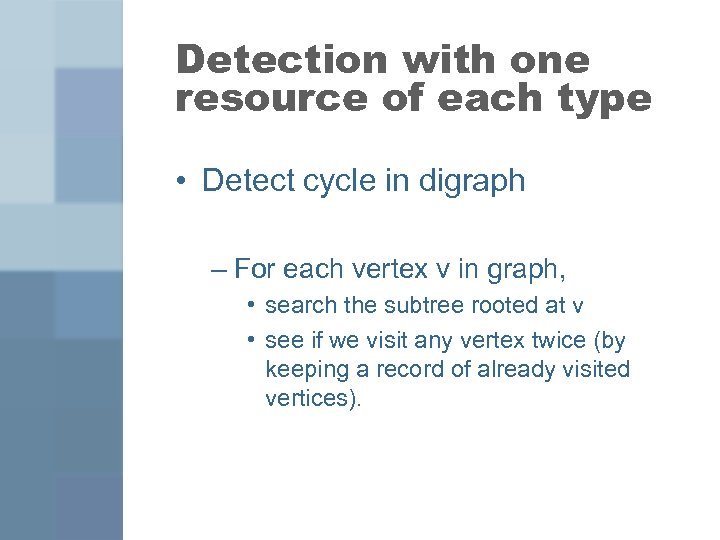Detection with one resource of each type • Detect cycle in digraph – For each vertex v in graph, • search the subtree rooted at v • see if we visit any vertex twice (by keeping a record of already visited vertices).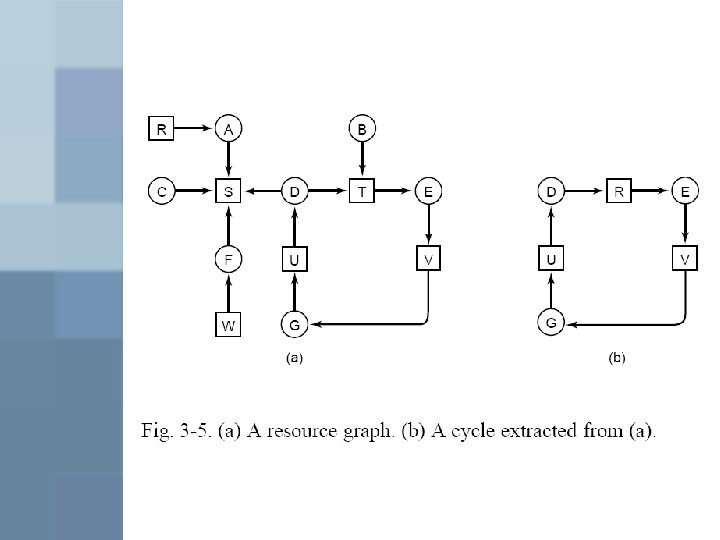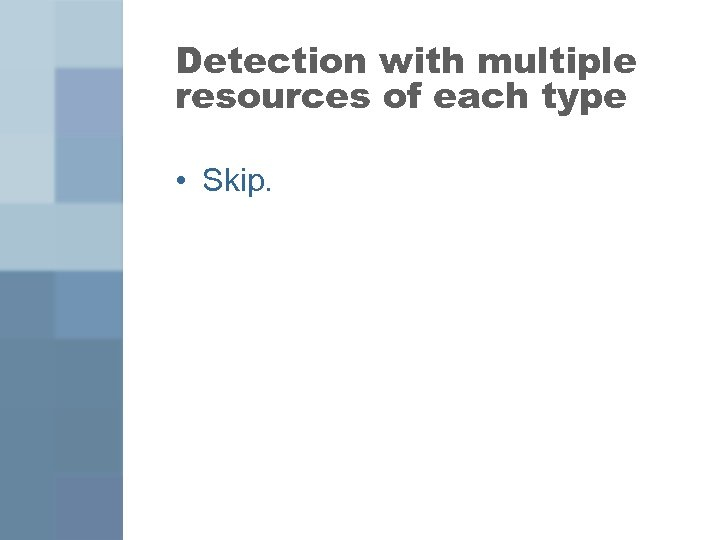Detection with multiple resources of each type • Skip.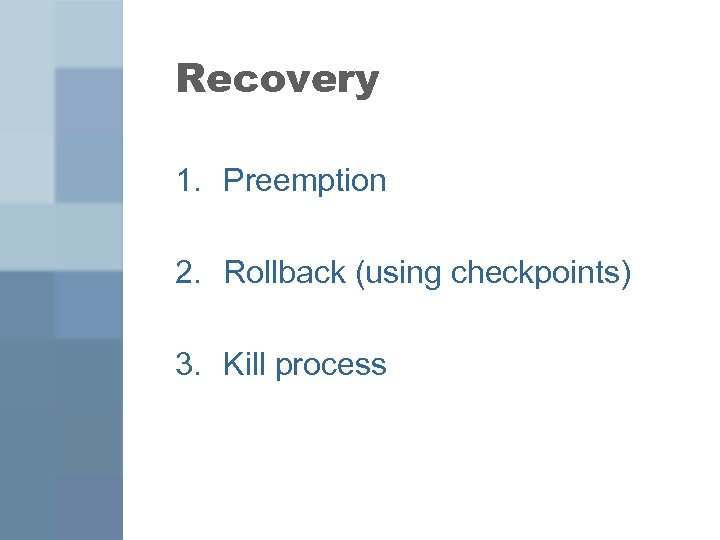Recovery 1. Preemption 2. Rollback (using checkpoints) 3. Kill process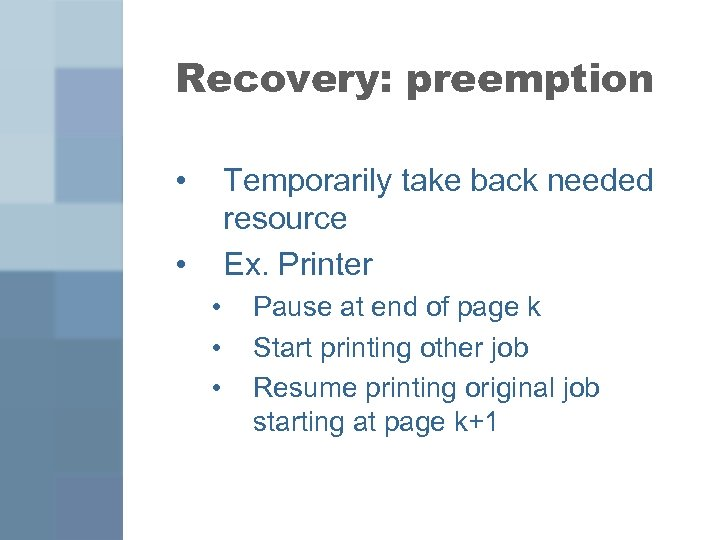Recovery: preemption • Temporarily take back needed resource Ex. Printer • • Pause at end of page k Start printing other job Resume printing original job starting at page k+1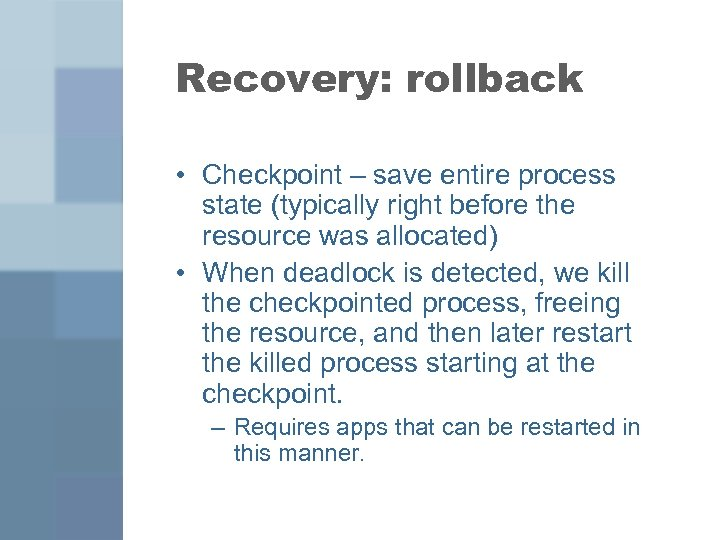Recovery: rollback • Checkpoint – save entire process state (typically right before the resource was allocated) • When deadlock is detected, we kill the checkpointed process, freeing the resource, and then later restart the killed process starting at the checkpoint. – Requires apps that can be restarted in this manner.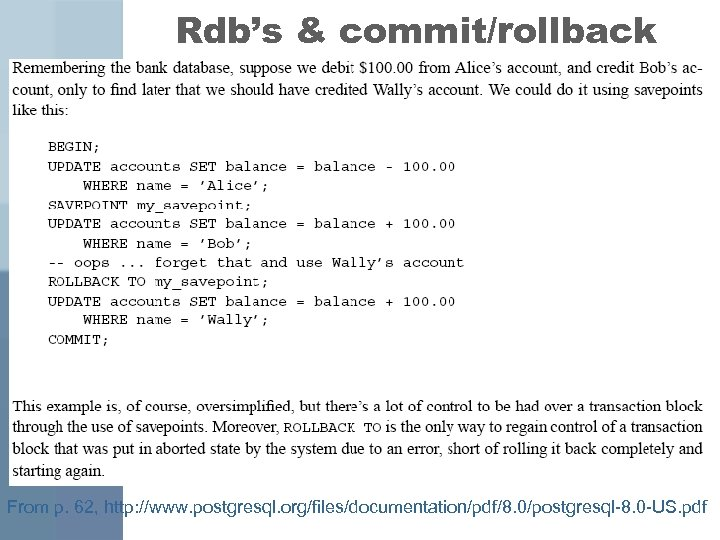Rdb’s & commit/rollback From p. 62, http: //www. postgresql. org/files/documentation/pdf/8. 0/postgresql-8. 0 -US. pdf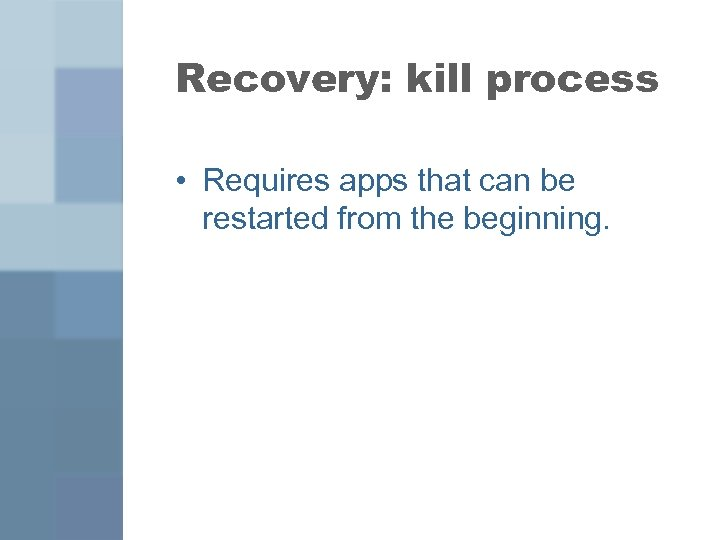Recovery: kill process • Requires apps that can be restarted from the beginning.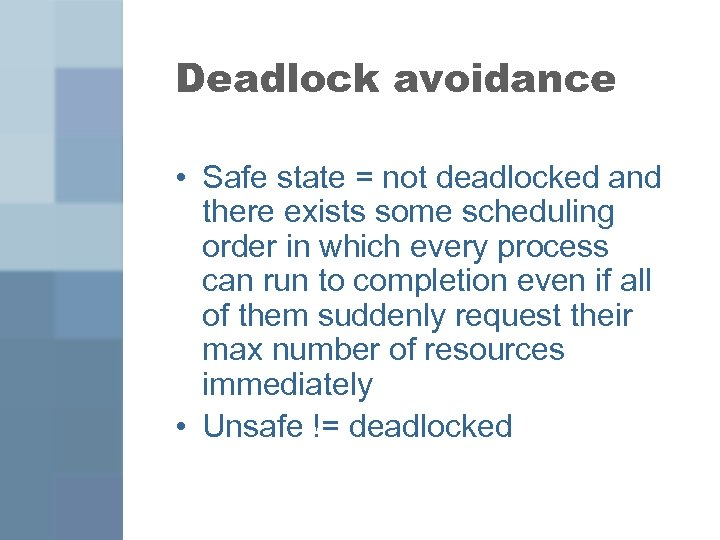Deadlock avoidance • Safe state = not deadlocked and there exists some scheduling order in which every process can run to completion even if all of them suddenly request their max number of resources immediately • Unsafe != deadlocked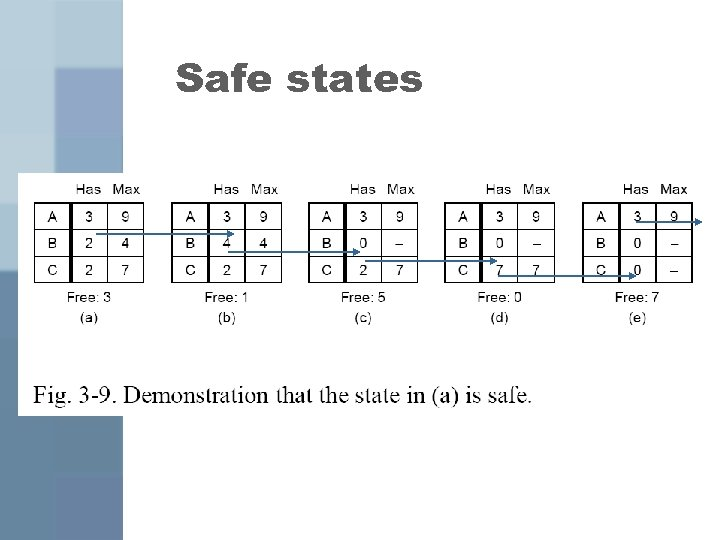Safe states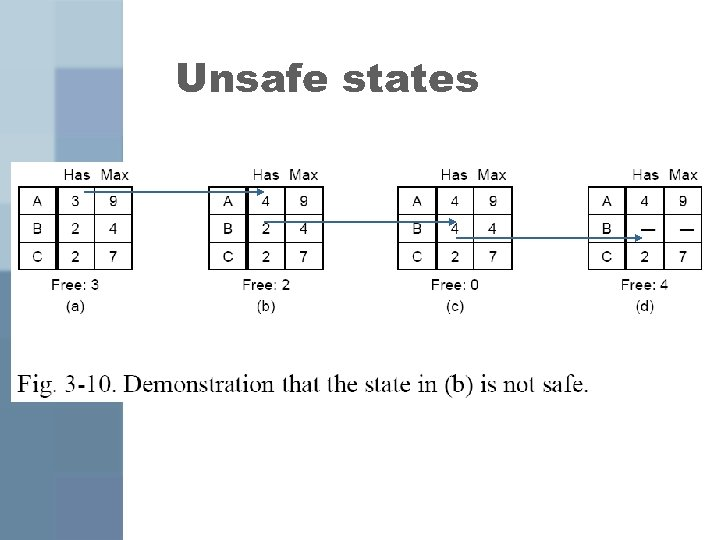Unsafe states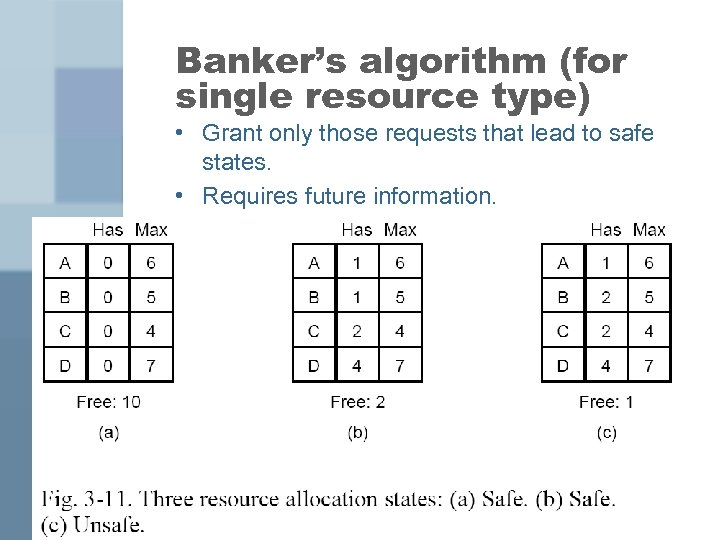Banker’s algorithm (for single resource type) • Grant only those requests that lead to safe states. • Requires future information.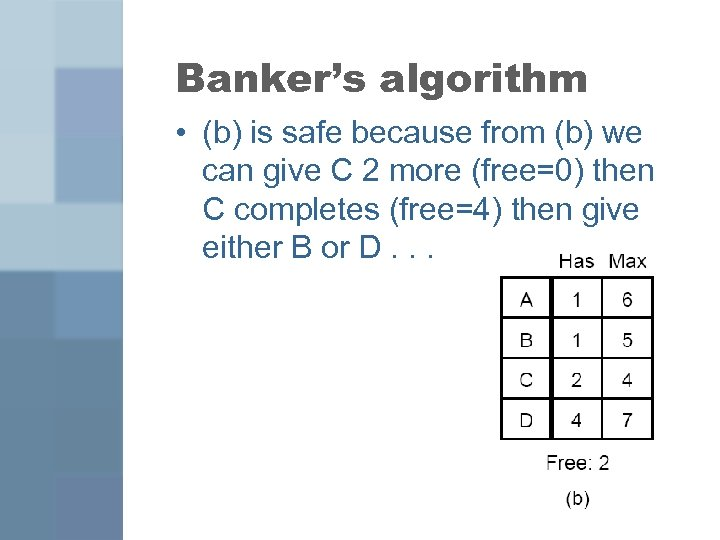Banker’s algorithm • (b) is safe because from (b) we can give C 2 more (free=0) then C completes (free=4) then give either B or D. . .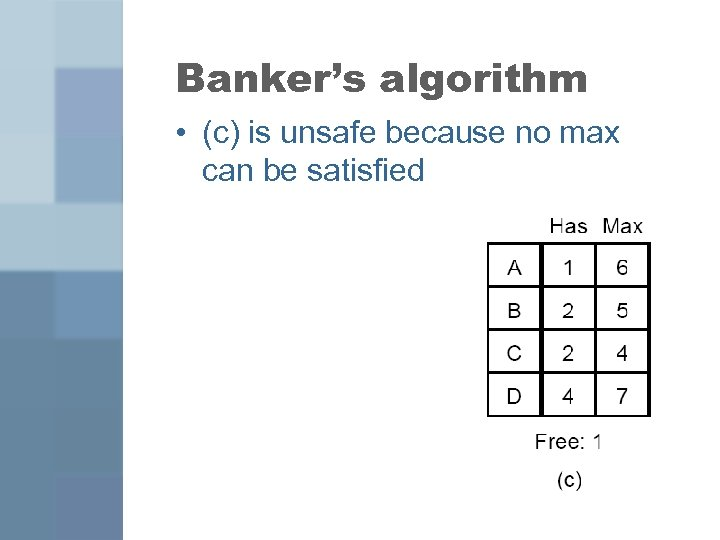Banker’s algorithm • (c) is unsafe because no max can be satisfied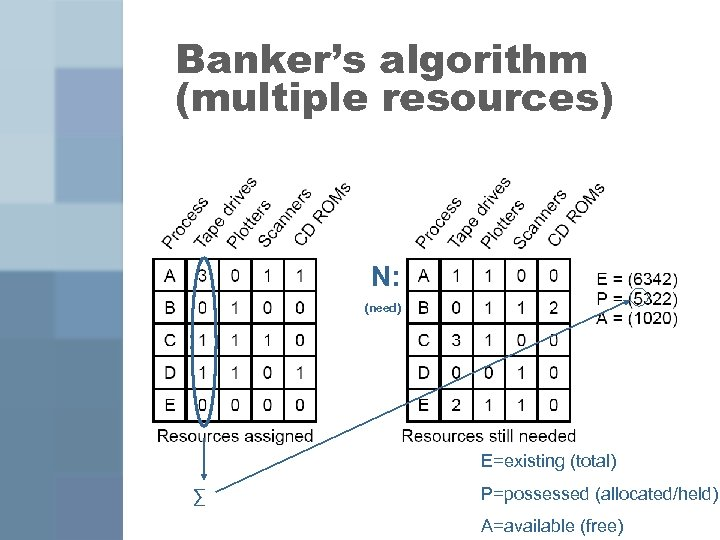Banker’s algorithm (multiple resources) N: (need) E=existing (total) ∑ P=possessed (allocated/held) A=available (free)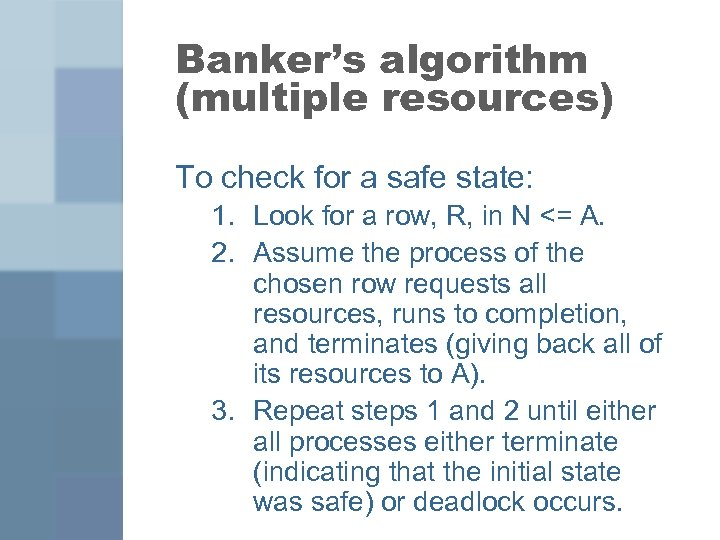Banker’s algorithm (multiple resources) To check for a safe state: 1. Look for a row, R, in N <= A. 2. Assume the process of the chosen row requests all resources, runs to completion, and terminates (giving back all of its resources to A). 3. Repeat steps 1 and 2 until either all processes either terminate (indicating that the initial state was safe) or deadlock occurs.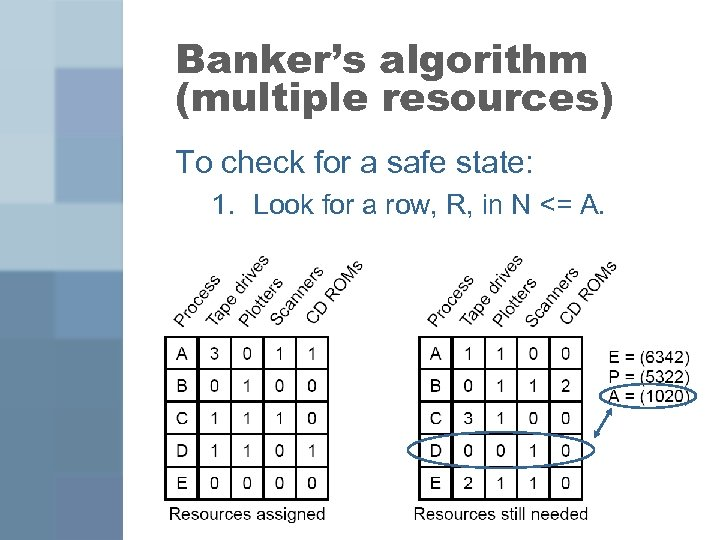Banker’s algorithm (multiple resources) To check for a safe state: 1. Look for a row, R, in N <= A.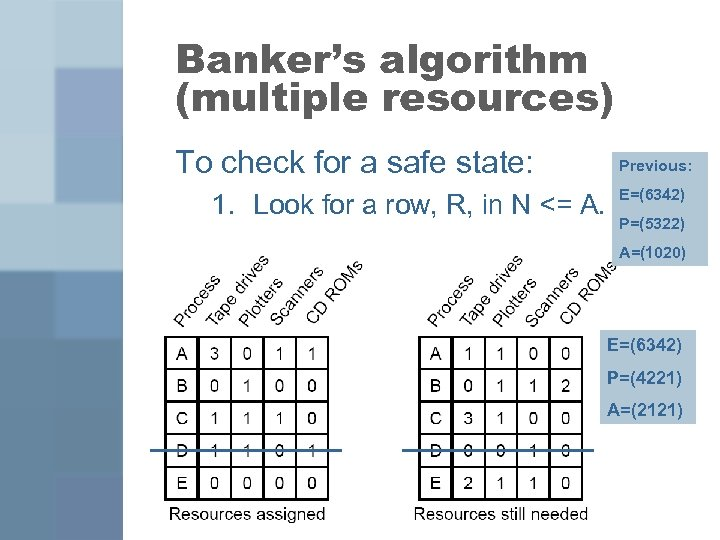Banker’s algorithm (multiple resources) To check for a safe state: 1. Look for a row, R, in N <= A. Previous: E=(6342) P=(5322) A=(1020) E=(6342) P=(4221) A=(2121)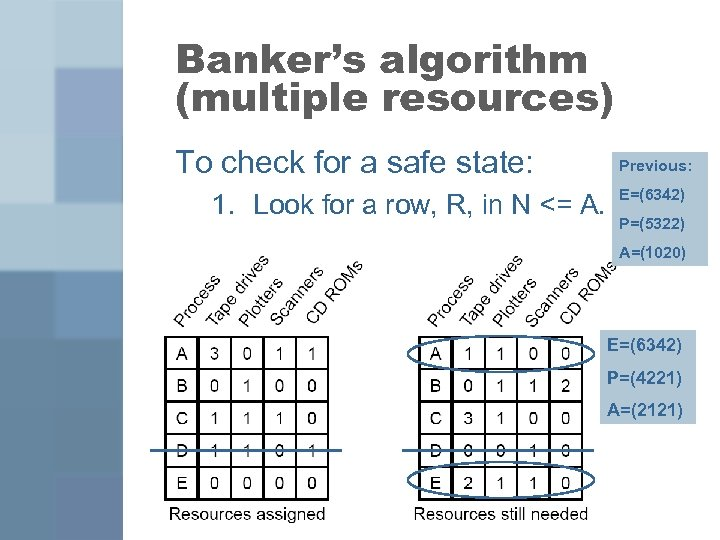Banker’s algorithm (multiple resources) To check for a safe state: 1. Look for a row, R, in N <= A. Previous: E=(6342) P=(5322) A=(1020) E=(6342) P=(4221) A=(2121)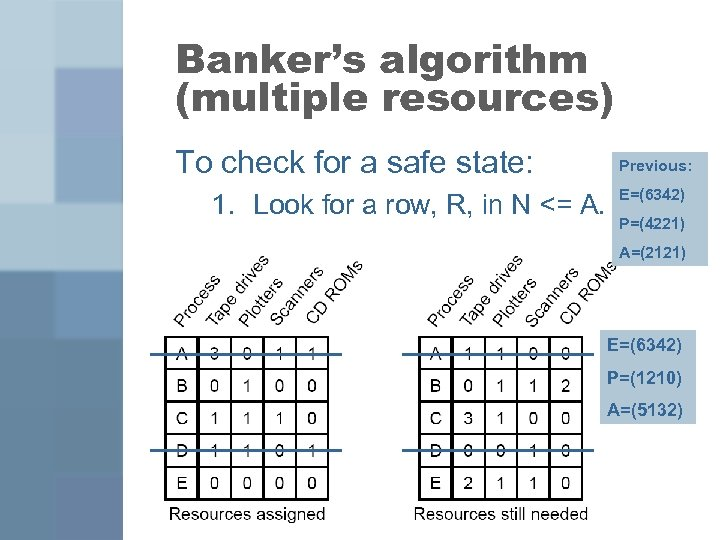Banker’s algorithm (multiple resources) To check for a safe state: 1. Look for a row, R, in N <= A. Previous: E=(6342) P=(4221) A=(2121) E=(6342) P=(1210) A=(5132)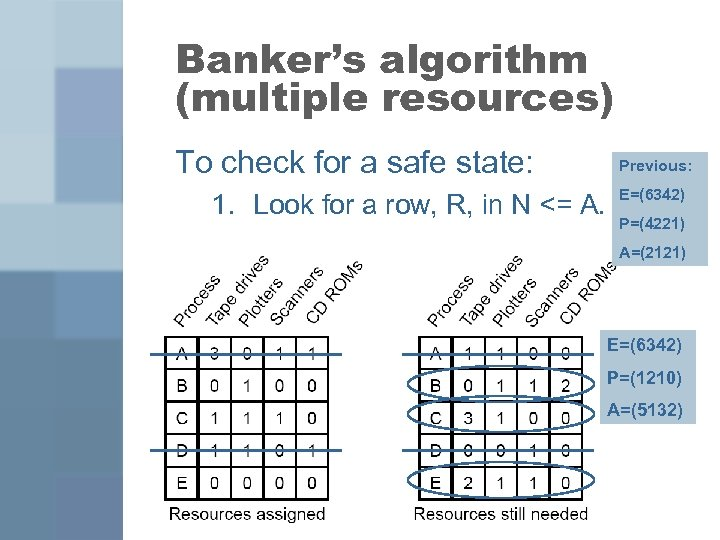Banker’s algorithm (multiple resources) To check for a safe state: 1. Look for a row, R, in N <= A. Previous: E=(6342) P=(4221) A=(2121) E=(6342) P=(1210) A=(5132)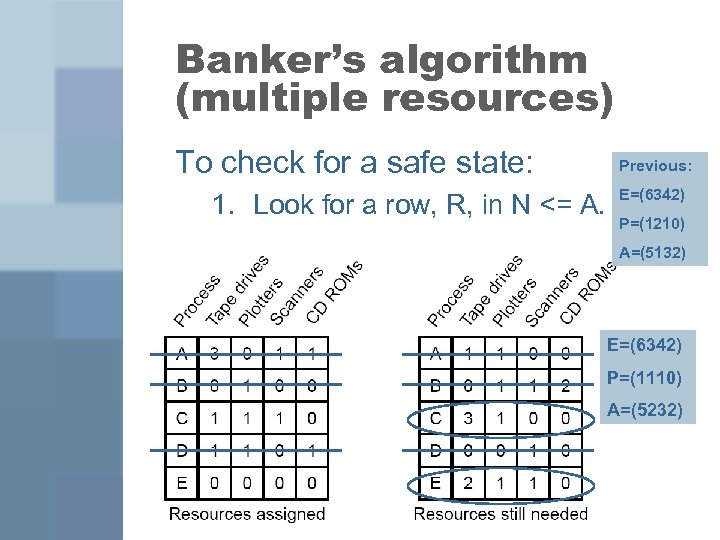Banker’s algorithm (multiple resources) To check for a safe state: 1. Look for a row, R, in N <= A. Previous: E=(6342) P=(1210) A=(5132) E=(6342) P=(1110) A=(5232)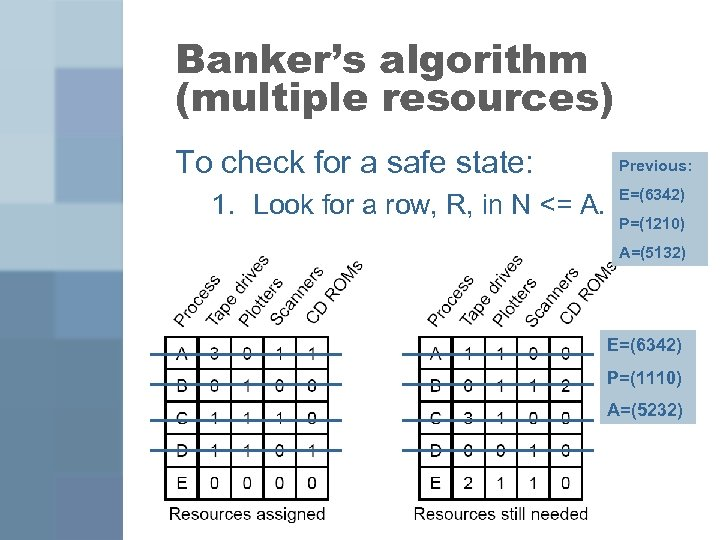Banker’s algorithm (multiple resources) To check for a safe state: 1. Look for a row, R, in N <= A. Previous: E=(6342) P=(1210) A=(5132) E=(6342) P=(1110) A=(5232)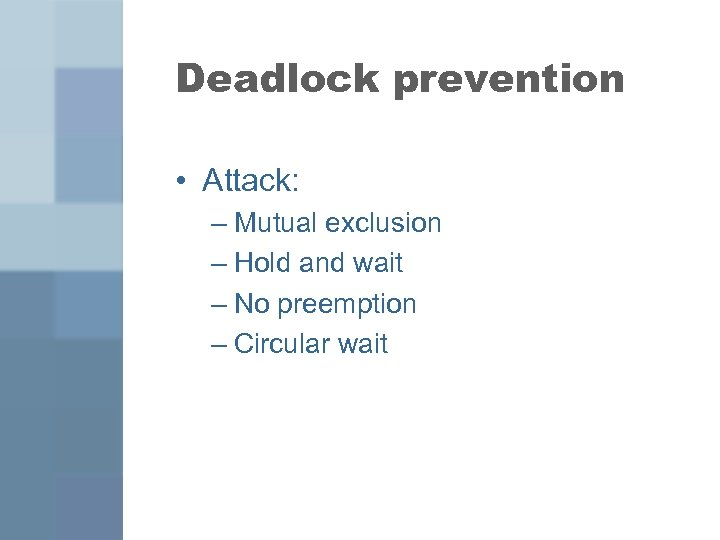Deadlock prevention • Attack: – Mutual exclusion – Hold and wait – No preemption – Circular wait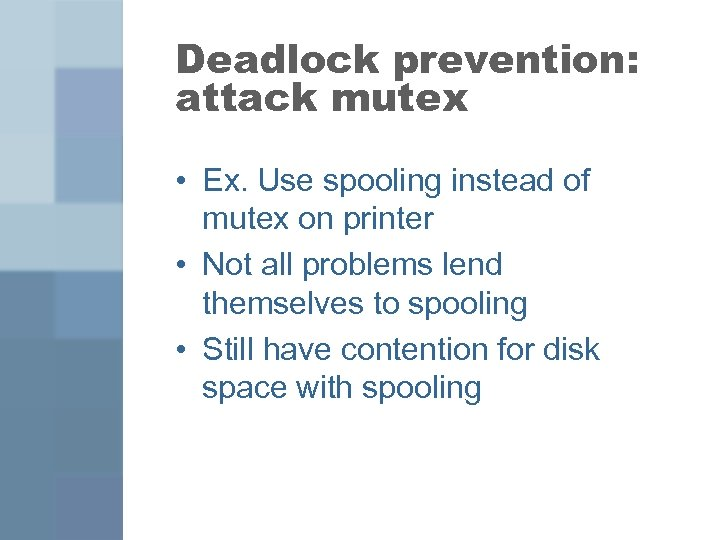Deadlock prevention: attack mutex • Ex. Use spooling instead of mutex on printer • Not all problems lend themselves to spooling • Still have contention for disk space with spooling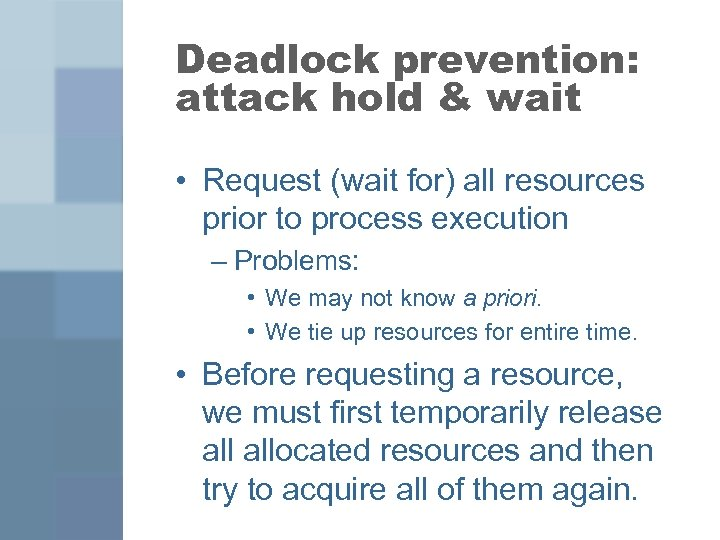Deadlock prevention: attack hold & wait • Request (wait for) all resources prior to process execution – Problems: • We may not know a priori. • We tie up resources for entire time. • Before requesting a resource, we must first temporarily release allocated resources and then try to acquire all of them again.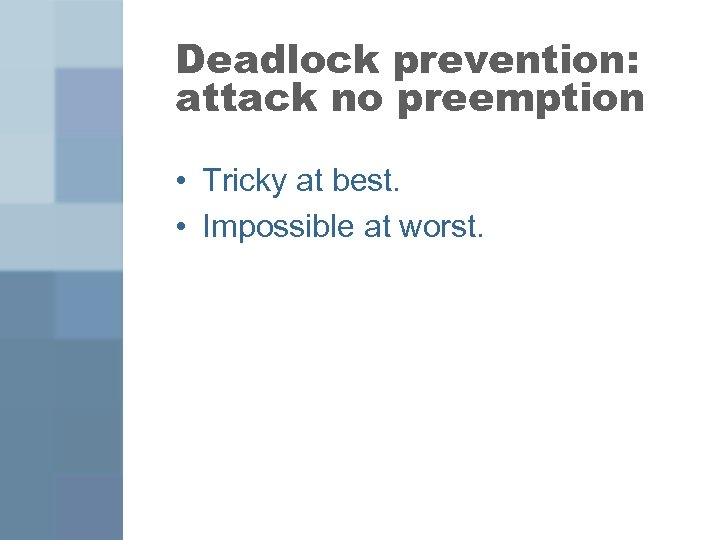Deadlock prevention: attack no preemption • Tricky at best. • Impossible at worst.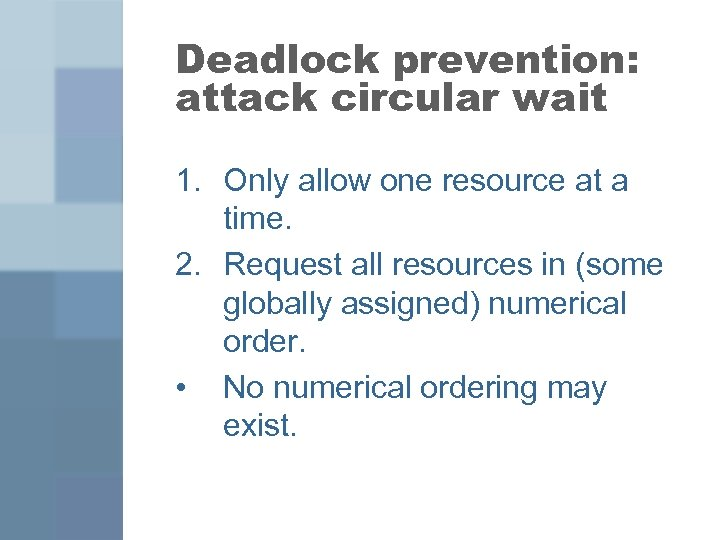Deadlock prevention: attack circular wait 1. Only allow one resource at a time. 2. Request all resources in (some globally assigned) numerical order. • No numerical ordering may exist.